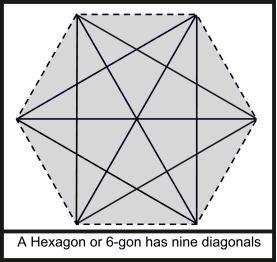Competitions

# Diagonal

The number of diagonals of an n-gon is not less than N. What is the minimum possible value of n?#### Input

Contains less than 1001 lines. Each line contains a positive integer N (N1015) that indicates the minimum possible number of diagonals. Input is terminated by a line with one 0 that should not be processed.

#### Output

For each test case produce one line that contains serial number and the minimum possible value for n (number of sides).

Time limit 1 second
Memory limit 128 MiB
Input example #1
10
100
1000
0

Output example #1
Case 1: 7
Case 2: 16
Case 3: 47